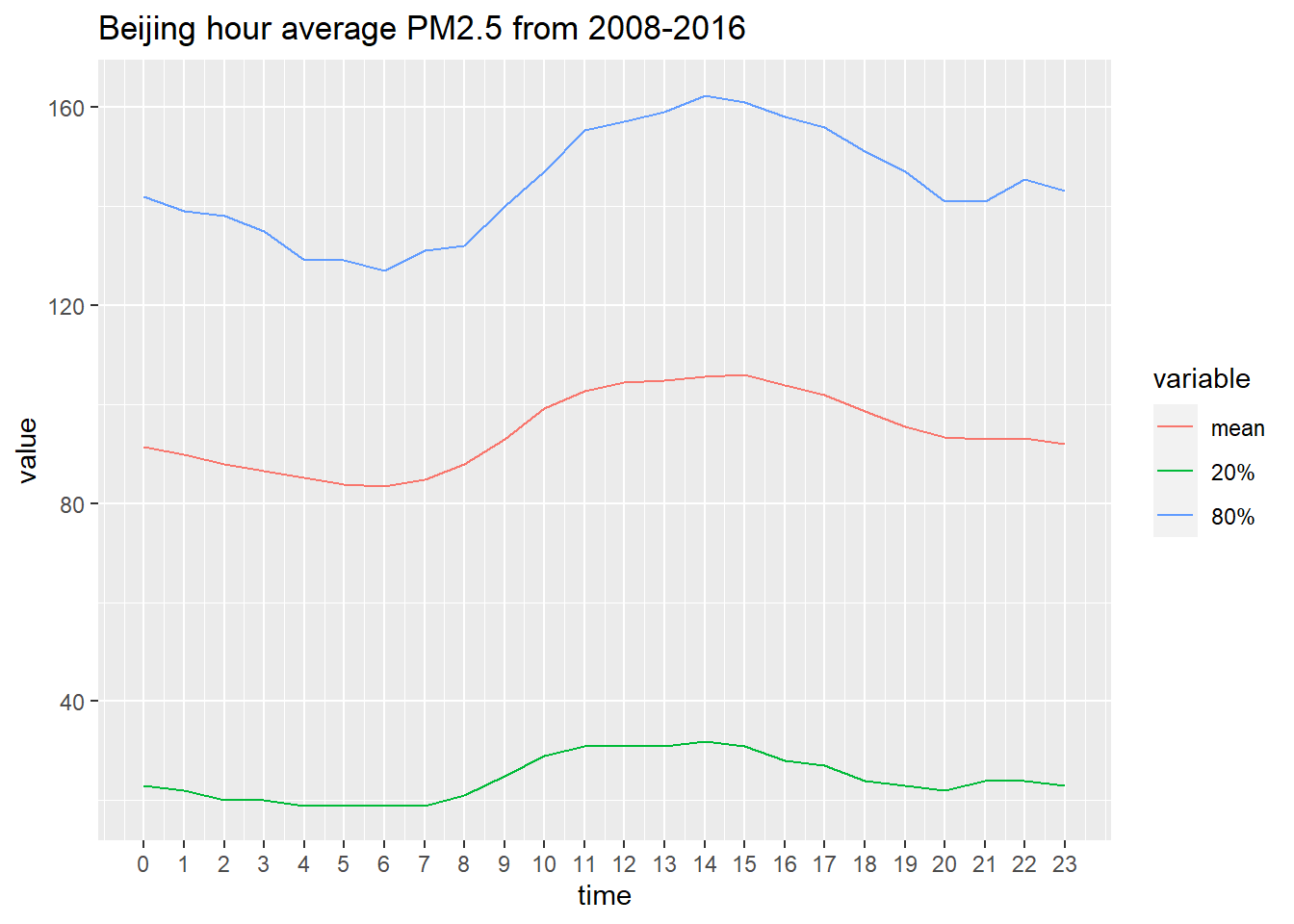The time-varying (longitudinal) characteristics of large information flows represent a special case of the complexity, dynamic and multi-scale nature of big biomedical data that we discussed in the DSPA Motivation section. Previously, in Chapter 3, we saw space-time (4D) functional magnetic resonance imaging (fMRI) data, and in Chapter 15 we discussed streaming data, which also has a natural temporal dimension.

In this Chapter, we will expand our predictive data analytic strategies specifically for analyzing longitudinal data. We will interrogate datasets that track the same type of information, for same subjects, units or locations, over a period of time. Specifically, we will present time series analysis, forecasting using autoregressive integrated moving average (ARIMA) models, structural equation models (SEM), and longitudinal data analysis via linear mixed models.

Note: This Chapter is purposely divided in two separate sections to reduce the page loading time, stratify the extensive computational tasks, and improve the user experiences. The separate RNN/LSTM section is available here, and it includes:

• Recurrent Neural Networks (RNN)
• Tensor Format Representation
• Simulated RNN case-study
• Climate Data Study
• Keras-based Multi-covariate LSTM Time-series Analysis and Forecasting.

# 1 Time series analysis

Time series analysis relies on models like ARIMA (Autoregressive integrated moving average) that utilize past longitudinal information to predict near future outcomes. Time series data tent to track univariate, sometimes multivariate, processes over a continuous time interval. The stock market, e.g., daily closing value of the Dow Jones Industrial Average index, or electroencephalography (EEG) data provide examples of such longitudinal datasets (time series).

The basic concepts in time series analysis include:

• The characteristics of (second-order) stationary time series (e.g., first two moments are stable over time) do not depend on the time at which the series process is observed.
• Differencing – a transformation applied to time-series data to make it stationary. Differences between consecutive time-observations may be computed by $$\displaystyle y_{t}'=y_{t}-y_{t-1}$$. Differencing removes the level changes in the time series, eliminates trend, reduces seasonality, and stabilizes the mean of the time series. Differencing the time series repeatedly may yield a stationary time series. For example, a second order differencing: \displaystyle {\begin{aligned}y_{t}^{''}&=y_{t}'-y_{t-1}'\\&=(y_{t}-y_{t-1})-(y_{t-1}-y_{t-2})\\&=y_{t}-2y_{t-1}+y_{t-2}\end{aligned}}.
• Seasonal differencing is computed as a difference between one observation and its corresponding observation in the previous epoch, or season (e.g., annually, there are $$m=4$$ seasons), like in this example: $\displaystyle y_{t}'''=y_{t}-y_{t-m}\quad {\text{where }}m={\text{number of seasons}}.$ The differenced data may then be used to estimate an ARMA model.

We will use the Beijing air quality PM2.5 dataset as an example to demonstrate the analysis process. This dataset measures air pollutants - PM2.5 particles in micrograms per cubic meter during the past 9 years (2008-2016). It measures the hourly average of the number of particles that are of size 2.5 microns (PM2.5) once per hour in Beijing, China.

Let’s first import the dataset into R.

beijing.pm25<-read.csv("https://umich.instructure.com/files/1823138/download?download_frd=1")
summary(beijing.pm25)
##      Index           Site            Parameter          Date..LST.
##  Min.   :    1   Length:69335       Length:69335       Length:69335
##  1st Qu.:17335   Class :character   Class :character   Class :character
##  Median :34668   Mode  :character   Mode  :character   Mode  :character
##  Mean   :34668
##  3rd Qu.:52002
##  Max.   :69335
##       Year          Month             Day             Hour
##  Min.   :2008   Min.   : 1.000   Min.   : 1.00   Min.   : 0.0
##  1st Qu.:2010   1st Qu.: 4.000   1st Qu.: 8.00   1st Qu.: 5.5
##  Median :2012   Median : 6.000   Median :16.00   Median :11.0
##  Mean   :2012   Mean   : 6.407   Mean   :15.73   Mean   :11.5
##  3rd Qu.:2014   3rd Qu.: 9.000   3rd Qu.:23.00   3rd Qu.:17.5
##  Max.   :2016   Max.   :12.000   Max.   :31.00   Max.   :23.0
##      Value           Duration           QC.Name
##  Min.   :-999.00   Length:69335       Length:69335
##  1st Qu.:  22.00   Class :character   Class :character
##  Median :  63.00   Mode  :character   Mode  :character
##  Mean   :  24.99
##  3rd Qu.: 125.00
##  Max.   : 994.00

The Value column records PM2.5 AQI (Air Quality Index) for the past 8 years. We observe that there are some missing data in the Value column. By looking at the QC.Name column, we only have about 6.5% (4,408 observations) missing values. One way of solving the missingness is to replace them by the corresponding variable mean.

beijing.pm25[beijing.pm25$Value==-999, 9]<-NA beijing.pm25[is.na(beijing.pm25$Value), 9]<-floor(mean(beijing.pm25$Value, na.rm = T)) Here we first reassign the missing values into NA labels. Then we replace all NA labels with the mean computed using all non-missing observations. Note that the floor() function casts the arithmetic averages as integer numbers, which is needed as AQI values are expected to be whole numbers. Now, let’s observe the trend of hourly average PM2.5 across one day. You can see a significant pattern: The PM2.5 level peeks in the afternoons and is the lowest in the early mornings and exhibits approximate periodic boundary conditions (these patterns oscillate daily). require(ggplot2) id = 1:nrow(beijing.pm25) mat = matrix(0,nrow=24,ncol=3) stat = function(x){ c(mean(beijing.pm25[iid,"Value"]),quantile(beijing.pm25[iid,"Value"],c(0.2,0.8))) } for (i in 1:24){ iid = which(id%%24==i-1) mat[i,] = stat(iid) } mat <- as.data.frame(mat) colnames(mat) <- c("mean","20%","80%") mat$time = c(15:23,0:14)
require(reshape2)
dt <- melt(mat,id="time")
colnames(dt)
##  "time"     "variable" "value"
ggplot(data = dt,mapping = aes(x=time,y=value,color = variable))+geom_line()+
scale_x_continuous(breaks = 0:23)+ggtitle("Beijing hour average PM2.5 from 2008-2016")Are there any daily or monthly trends? We can start the data interrogation by building an ARIMA model and examining detailed patterns in the data.

## 1.1 Step 1: Plot time series

To begin with, we can visualize the overall trend by plotting PM2.5 values against time. This can be achieved using the plyr package.

library(plyr)
ts<-ts(beijing.pm25\$Value, start=1, end=69335, frequency=1)
ts.plot(ts)